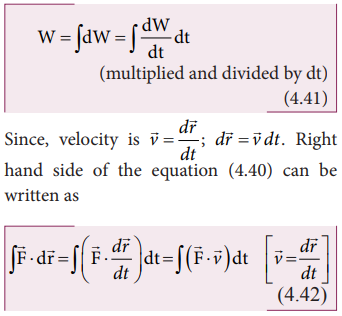Home | | Physics 11th std | Relation between power and velocity

# Relation between power and velocity

Physics : Work, Energy and Power

Relation between power and velocity

The work done by a forcefor a displacement disLeft hand side of the equation (4.40) can be written asSubstituting equation (4.41) and equation (4.42) in equation (4.40), we getThis relation is true for any arbitrary value of dt. This implies that the term within the bracket must be equal to zero, i.e.,## Solved Example Problems for Relation between power and velocity

### Example 4.19

A vehicle of mass 1250 kg is driven with an acceleration 0.2 ms-2 along a straight level road against an external resistive force 500 N.Calculate the power delivered by the vehicle’s engine if the velocity of the vehicle is 30 m s-1 .

### Solution

The vehicle’s engine has to do work against resistive force and make vechile to move with an acceleration. Therefore, power delivered by the vehicle engine is

Study Material, Lecturing Notes, Assignment, Reference, Wiki description explanation, brief detail
11th Physics : UNIT 4 : Work, Energy and Power : Relation between power and velocity |